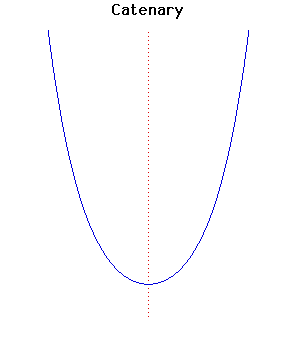# Curves

### CatenaryCartesian equation:
$y = a \cosh(x/a)$

### Description

The catenary is the shape of a perfectly flexible chain suspended by its ends and acted on by gravity. Its equation was obtained by Leibniz, Huygens and Johann Bernoulli in 1691. They were responding to a challenge put out by Jacob Bernoulli to find the equation of the 'chain-curve'.

Huygens was the first to use the term catenary in a letter to Leibniz in 1690 and David Gregory wrote a treatise on the catenary in 1690. Jungius (1669) disproved Galileo's claim that the curve of a chain hanging under gravity would be a parabola.

The catenary is the locus of the focus of a parabola rolling along a straight line.

The catenary is the evolute of the tractrix. It is the locus of the mid-point of the vertical line segment between the curves $e^{x}$ and $e^{-x}$.

Euler showed in 1744 that a catenary revolved about its asymptote generates the only minimal surface of revolution.

### Associated Curves

Definitions of the Associated curves